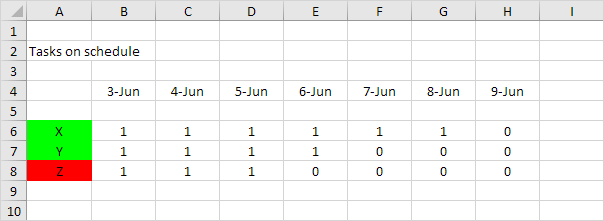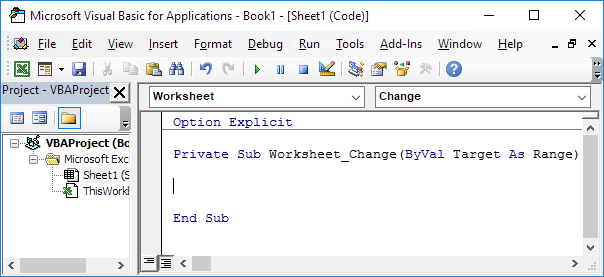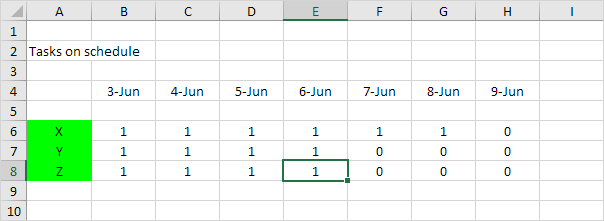Below we will look at a program in Excel VBA that sets the background color of tasks that are on schedule to green, and sets the background color of tasks that are behind schedule to red.

Situation:

On Sheet1 we have three tasks (X, Y, Z). A "1" indicates that a task has been completed. A task is on schedule if a "1" exist in every column up to and including today's date. Today it's 6-Jun. Tasks X and Y are on schedule. Task Z is behind schedule (no "1" in cell E8).Create a Worksheet Change Event. Code added to the Worksheet Change Event will be executed by Excel VBA when you change a cell on a worksheet.

1. Open the Visual Basic Editor.

2. Double click on Sheet1 (Sheet1) in the Project Explorer.

3. Choose Worksheet from the left drop-down list. Choose Change from the right drop-down list.Add the following code lines to the Worksheet Change Event:

4. Declare two variables of type Integer.

Dim i As Integer, j As Integer

5. Add a Do While Loop.

Do While Cells(6 + i, 1).Value <> ""

i = i + 1
Loop

Explanation: For i = 0, Excel VBA checks task X, for i = 1, task Y, etc. Excel VBA leaves the Do While loop when Cells(6 + i, 1).Value is empty (no more tasks to check).

Add the following code lines (at 6, 7 and 8) to the Do While Loop.

6. Initialize the variable j with value 0.

j = 0

7. Set the background color of a task to green assuming that a task is on schedule (this is not necessarily true of course).

Cells(6 + i, 1).Interior.ColorIndex = 4

8. Add another Do While Loop.

Do While Cells(4, 2 + j).Value <= Date
If Cells(6 + i, 2 + j).Value = 0 Then Cells(6 + i, 1).Interior.ColorIndex = 3
j = j + 1
Loop

Note: the Date function returns today's date.

Explanation: For i = 0, j = 0, Excel VBA checks cell B6. For i = 0, j = 1, Excel VBA checks cell C6, etc. A task is on schedule if a "1" exists in every column up to and including today's date. If Excel VBA finds a "0", it sets the background color of the task to red.

9. Test the program. For example, indicate that task Z on 6-Jun has been completed. As a result, task Z will automatically turn green.Go to Next Chapter: Events